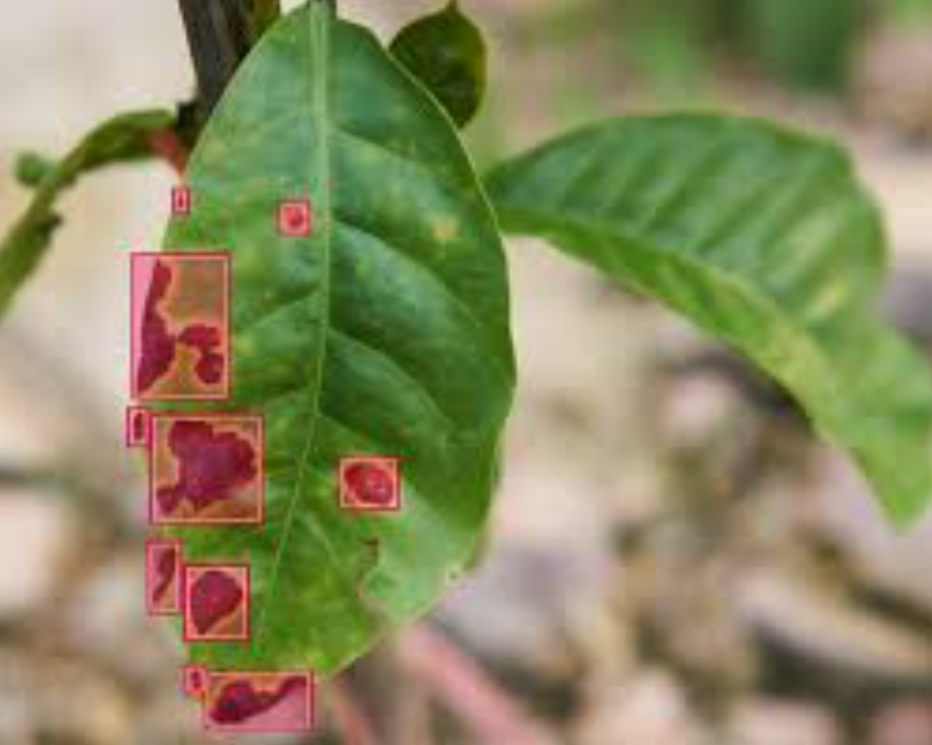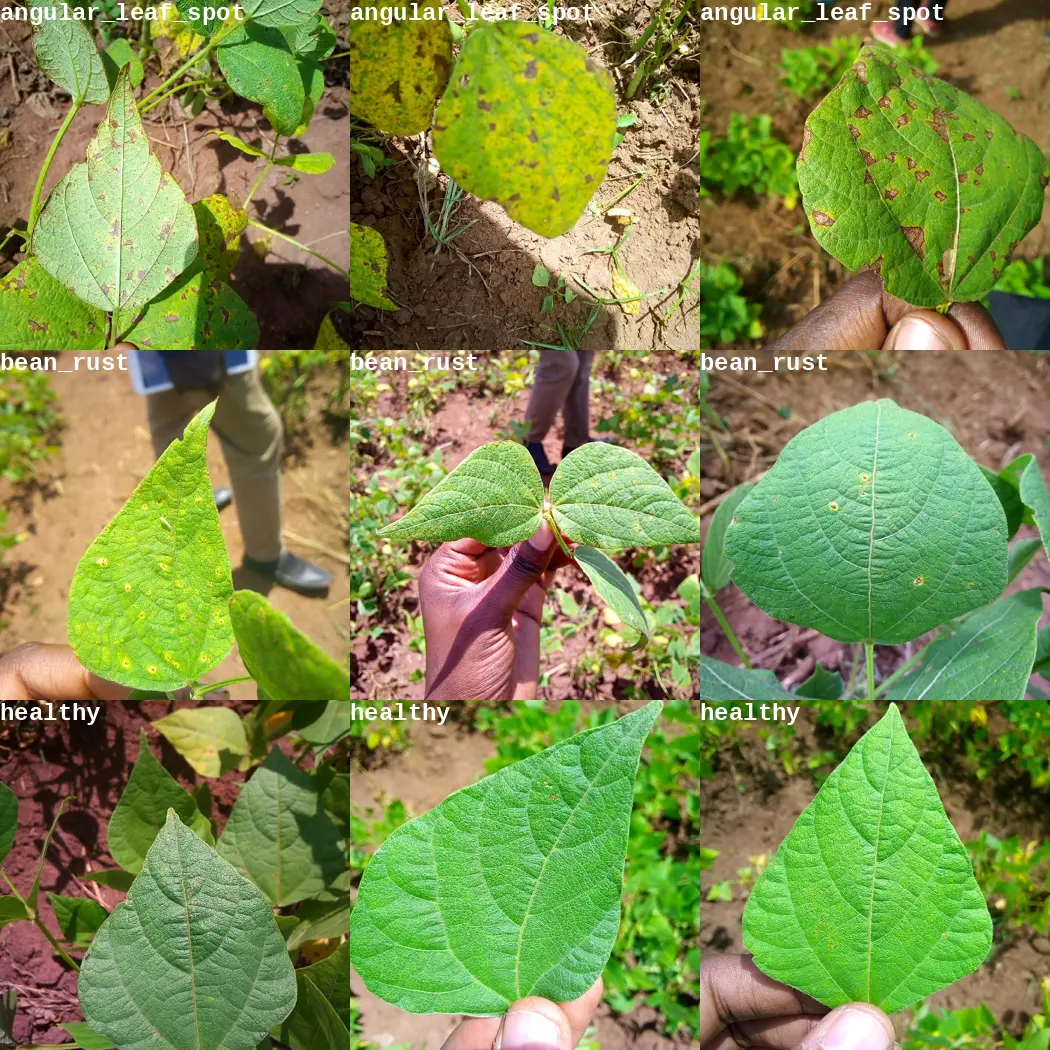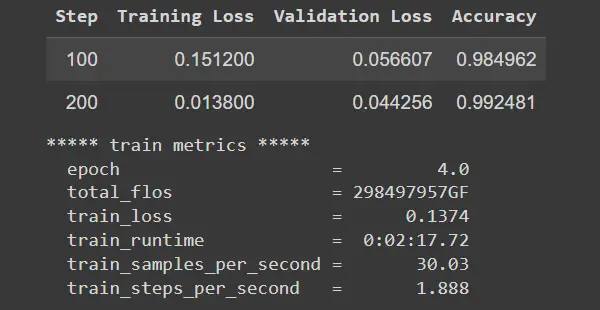Black Friday Sale is Here! Get 35% Discounts on Subscription & Data Credits- Know More

# Vision Transformers For Identification of Healthy and Diseased Leaves - Tutorial

This blog shows how we can utilize a vision transformer for an Image classification tasks. Particularly, we use Leaf disease classification dataset.Vision Transformers For Identification of Healthy and Diseased Leaves - Tutorial

Vision Transformers, also known as ViT, was introduced in a paper by the Google Brain team in late October 2020. To understand how ViT works, you should already know how Transformers work and the problems they help solve.

You can refer to our previous blog post for a quick understanding of VITs and their work.

This blog aims to utilize Vision Transformers for a leaf-disease classification task. We will understand an entire pipeline of how we can load a pre-trained ViT, fine-tune it, and get results.

For our fine-tuning VIT Tutorial, we have used a leaf-disease classification dataset called beans-dataset, which is available here.

The dataset contains about 1,000 train images and around 130 test images. We have kept the data small to focus more on how to implement the fine-tuning and not spend too much time on hyper-parameter tuning.

The data used is in the following format:

1. image_file_path: a string file path to an image.
2. image: A PIL.Image.Image object containing the image.
3. labels: an int classification label.

There are 3 classes which are to be classified, which include:

1. Class 0: Angular Bean Spot
2. Class 1: Bean Rust
3. Class 2: HealthyFigure: Sample Images From Dataset

The below tutorial can be utilized for your custom data as well. For gathering labelled data with utmost accuracy, I recommend using Labeller Data annotation Platform.

### Hands-on For Tutorial

Before beginning to code, we look at the task's prerequisites.

Pre-requisite

To proceed further, one should be familiar with:

1. Python: All the below code will be written using Python.
2. Pytorch:  PyTorch, founded on the Torch library, is a machine learning framework utilized for tasks like computer vision and natural language processing.
3. Colab: Colab, short for Google Colaboratory, is a free cloud-based platform that provides access to GPUs and allows collaborative coding in Python.

Tutorial

We begin with installing the required libraries.

``````! pip install datasets transformers
! pip install -U accelerate
! pip install -U transformers``````

``````from datasets import load_dataset
ds``````

To better understand the data, we plot the data in grid form.

``````from transformers.utils.dummy_vision_objects import ImageGPTFeatureExtractor
import random
from PIL import ImageDraw, ImageFont, Image

def show_examples(ds, seed: int = 1234, examples_per_class: int = 3, size=(350, 350)):

w, h = size
labels = ds['train'].features['labels'].names
grid = Image.new('RGB', size=(examples_per_class * w, len(labels) * h))
draw = ImageDraw.Draw(grid)
font = ImageFont.truetype("/usr/share/fonts/truetype/liberation/LiberationMono-Bold.ttf", 24)

for label_id, label in enumerate(labels):

# Filter the dataset by a single label, shuffle it, and grab a few samples
ds_slice = ds['train'].filter(lambda ex: ex['labels'] == label_id).shuffle(seed).select(range(examples_per_class))

# Plot this label's examples along a row
for i, example in enumerate(ds_slice):
image = example['image']
idx = examples_per_class * label_id + i
box = (idx % examples_per_class * w, idx // examples_per_class * h)
grid.paste(image.resize(size), box=box)
draw.text(box, label, (255, 255, 255), font=font)

return grid

show_examples(ds, seed=random.randint(0, 1337), examples_per_class=3)``````

Now that we have a clear view of our image content and a deeper grasp of the problem we aim to address let's explore how to prepare these images for our model.

When training ViT models, specific image transformations are employed. Applying the wrong transformations to your image could make the model struggle to comprehend its visual input.

To ensure the correct application of these transformations, we'll utilize a ViTFeatureExtractor that is initialized with a configuration saved alongside the pretrained model we intend to use.

In our case, we'll be working with the google/vit-base-patch16-224-in21k model, so let's proceed to load its feature extractor.

``````from transformers import ViTFeatureExtractor
feature_extractor = ViTFeatureExtractor.from_pretrained(model_name_or_path)``````

Now that we know how to read in images and transform them into inputs, let's write a function that will put those two things together to process a single example from the dataset.

``````def process_example(example):
inputs = feature_extractor(example['image'], return_tensors='pt')
inputs['labels'] = example['labels']
return inputs``````

While we could call ds.map and apply this to every example at once, this can be very slow, especially if you use a larger dataset. Instead, we'll apply a transform to the dataset. Transforms are only applied to examples as you index them.

First, though, we'll need to update our last function to accept a batch of data, as that's what ds.with_transform expects.

``````ds = load_dataset('beans')

def transform(example_batch):
# Take a list of PIL images and turn them to pixel values
inputs = feature_extractor([x for x in example_batch['image']], return_tensors='pt')

# Don't forget to include the labels!
inputs['labels'] = example_batch['labels']
return inputs

prepared_ds = ds.with_transform(transform)``````

The data has been processed, and we're now poised to initiate the training pipeline. We'll employ a huggingface Trainer for this purpose, but there are a few preliminary tasks to tackle:

1. Create a collate function.
2. Specify an evaluation metric. Throughout the training, assessing the model's predictive accuracy is vital, necessitating the definition of a compute_metrics function to serve this purpose.
3. Load a pretrained checkpoint. We must load a pretrained checkpoint and ensure it's properly configured for training.
4. Establish the training configuration.

Once the model has undergone fine-tuning, we'll proceed to conduct a thorough evaluation on the validation data to confirm its ability to classify our images accurately.

Batches are coming in as lists of dicts, so we just unpack + stack those into batch tensors.

``````import torch

def collate_fn(batch):
return {
'pixel_values': torch.stack([x['pixel_values'] for x in batch]),
'labels': torch.tensor([x['labels'] for x in batch])
}``````

Here, we load the accuracy metric from datasets and then write a function that takes in a model prediction + computes the accuracy.

``````import numpy as np

def compute_metrics(p):
return metric.compute(predictions=np.argmax(p.predictions, axis=1), references=p.label_ids)``````

Now, we can bring in our pre-trained model. When we initialize it, we'll specify the number of labels so that the model sets up a classification head with the correct number of components.

``````from transformers import ViTForImageClassification

labels = ds['train'].features['labels'].names

model = ViTForImageClassification.from_pretrained(
model_name_or_path,
num_labels=len(labels),
id2label={str(i): c for i, c in enumerate(labels)},
label2id={c: str(i) for i, c in enumerate(labels)}
)``````

We're almost at the training stage! The final step before that is establishing the training configuration by defining Training Arguments.

Most of these configuration settings are straightforward. However, one that's especially important in this context is "remove_unused_columns=False."

Typically, it's set to "True" by default, which means that any features not utilized by the model's call function are discarded. This default behavior is useful in most cases as it simplifies the input for the model's call function.

However, in our situation, we require the unused features, especially 'image,' to generate 'pixel_values.'

``````# !pip install transformers[torch]
# !pip install accelerate -U

training_args = TrainingArguments(
output_dir="./vit-base-beans-demo-v5",
per_device_train_batch_size=16,
evaluation_strategy="steps",
num_train_epochs=4,
fp16=True,
save_steps=100,
eval_steps=100,
logging_steps=10,
learning_rate=2e-4,
save_total_limit=2,
remove_unused_columns=False,
push_to_hub=False,
report_to='tensorboard',
)``````

After setting the training configurations above, we can begin with our training.

``````from transformers import Trainer

trainer = Trainer(
model=model,
args=training_args,
data_collator=collate_fn,
compute_metrics=compute_metrics,
train_dataset=prepared_ds["train"],
eval_dataset=prepared_ds["validation"],
tokenizer=feature_extractor,
)``````

Lastly, we evaluate our model results.

``````train_results = trainer.train()
trainer.save_model()
trainer.log_metrics("train", train_results.metrics)
trainer.save_metrics("train", train_results.metrics)
trainer.save_state()``````Figure: Evaluation Results on Training Data

### Conclusion

In conclusion, this blog post explores the use of Vision Transformers (ViT) for the classification of leaf diseases. ViT, introduced by the Google Brain team in late 2020, leverages the power of transformers for solving visual tasks.

To provide a clear overview, the following key points are highlighted:

Dataset

The tutorial uses a leaf-disease classification dataset called "beans-dataset," containing approximately 1000 training images and 130 test images. The dataset comprises images with associated classification labels for Angular Bean Spot, Bean Rust, and Healthy.

Prerequisite

Before diving into the code, it's important to be familiar with Python, PyTorch (a machine learning framework), and Google Colaboratory (Colab), which is a cloud-based platform for collaborative Python coding.

Tutorial

The tutorial takes a step-by-step approach:

1. It starts by installing the necessary libraries and loading the dataset.
2. It visualizes the data in a grid format for better understanding.
3. The tutorial progresses to explain how to process and transform images for model input.
4. A transformation method is applied to the dataset to handle larger datasets efficiently, ensuring that transformations are only carried out when indexing examples.
5. The blog introduces the concept of the training pipeline, covering aspects such as collate functions, evaluation metrics, pretrained model loading, and training configuration settings.
6. The Trainer class from the Hugging Face Transformers library is utilized for training the model.
7. Training is conducted, and the results are evaluated to assess the model's performance.

The overall objective is to demonstrate the use of Vision Transformers for image classification, and the step-by-step guide provides insights into how to implement this in practice.

1.  What is Vission Transformer for Image Classification?

A Vision Transformer (ViT) is an architectural approach designed for classifying and working with images. ViT utilizes self-attention mechanisms to analyze and understand visual data.

The ViT architecture is structured around a sequence of transformer blocks, and each of these blocks is made up of two key components: a multi-head self-attention layer and a feed-forward layer.

2.  What is the difference between CNNs and Vision Transformers?

CNNs are a more established and well-understood architectural approach, making them more straightforward to learn, apply, and train compared to Transformers. CNNs rely on convolution, a technique that operates locally, focusing on a small region of an image at a time.

In contrast, Visual Transformers employ self-attention, a global mechanism that considers information from the entire image, thus offering a broader perspective.

Train Your Vision/NLP/LLM Models 10X Faster

Book our demo with one of our product specialist

Book a Demo